2022 NL Cy Young Odds
+180
1.8 to 1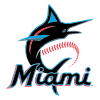Sandy Alcantara
27.2% implied probability

+550
5.5 to 1Corbin Burnes
11.7% implied probability

+650
6.5 to 1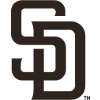Joe Musgrove
10.1% implied probability

+1500
15 to 1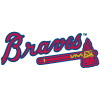Max Fried
4.8% implied probability

+1500
15 to 1Carlos Rodon
4.8% implied probability

+1500
15 to 1Zack Wheeler
4.8% implied probability

+2200
22 to 1Aaron Nola
3.3% implied probability

+2200
22 to 1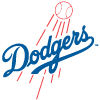Tony Gonsolin
3.3% implied probability

+5000
50 to 1Pablo Lopez
1.5% implied probability

+5000
50 to 1Josh Hader
1.5% implied probability

+6000
60 to 1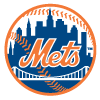Max Scherzer
1.2% implied probability

+6000
60 to 1Julio Urias
1.2% implied probability

+7000
70 to 1Logan Webb
1.1% implied probability

+7000
70 to 1Zac Gallen
1.1% implied probability

+7000
70 to 1Taijuan Walker
1.1% implied probability

No changes have been recorded yet.
+7000
70 to 1Yu Darvish
1.1% implied probability

+7000
70 to 1Kyle Wright
1.1% implied probability

+7000
70 to 1Clayton Kershaw
1.1% implied probability

+8000
80 to 1Sean Manaea
0.9% implied probability

+8000
80 to 1Eric Lauer
0.9% implied probability

+8000
80 to 1MacKenzie Gore
0.9% implied probability

+9000
90 to 1Tyler Anderson
0.8% implied probability

+10000
100 to 1Brandon Woodruff
0.8% implied probability

+10000
100 to 1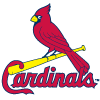Miles Mikolas
0.8% implied probability

+10000
100 to 1Ian Anderson
0.8% implied probability

+10000
100 to 1Freddy Peralta
0.8% implied probability

+10000
100 to 1Adam Wainwright
0.8% implied probability

+10000
100 to 1Walker Buehler
0.8% implied probability

+10000
100 to 1Carlos Carrasco
0.8% implied probability

+10000
100 to 1Chris Bassitt
0.8% implied probability

+12000
120 to 1Merrill Kelly
0.6% implied probability

+12000
120 to 1Jacob deGrom
0.6% implied probability

+12000
120 to 1Jesus Luzardo
0.6% implied probability

+12000
120 to 1Tylor Megill
0.6% implied probability

+15000
150 to 1Trevor Rogers
0.5% implied probability

+15000
150 to 1Kyle Gibson
0.5% implied probability

+15000
150 to 1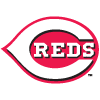Tyler Mahle
0.5% implied probability

+25000
250 to 1Ranger Suarez
0.3% implied probability

+25000
250 to 1Mike Clevinger
0.3% implied probability

+25000
250 to 1Andrew Heaney
0.3% implied probability

+25000
250 to 1Charlie Morton
0.3% implied probability

+25000
250 to 1Madison Bumgarner
0.3% implied probability

+25000
250 to 1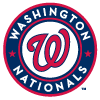Stephen Strasburg
0.3% implied probability

+25000
250 to 1Luis Castillo
0.3% implied probability

+25000
250 to 1Jack Flaherty
0.3% implied probability

+25000
250 to 1Blake Snell
0.3% implied probability

+25000
250 to 1Alex Cobb
0.3% implied probability

+25000
250 to 1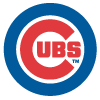Kyle Hendricks
0.3% implied probability

+50000
500 to 1Steven Matz
0.2% implied probability

+50000
500 to 1Anthony DeSclafani
0.2% implied probability

+50000
500 to 1Patrick Corbin
0.2% implied probability

+50000
500 to 1Marcus Stroman
0.2% implied probability

+50000
500 to 1Dustin May
0.2% implied probability

+50000
500 to 1Mike Soroka
0.2% implied probability

+50000
500 to 1Dinelson Lamet
0.2% implied probability

+50000
500 to 1German Marquez
0.2% implied probability